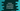# Python Tutorial : Part 6 : Python List module### Python list :

Sequence is the basic data structure used in python. There are 6 sequence types : strings, Unicode Strings, lists,tuples, buffers and xrange objects. The list is a most versatile datatype among these. In this tutorial, we will learn different operations we can perform on a list.

### Create a list :

List items are written within a square bracket [.] , separating each values by a comma. A list can have any number of items and it is not required that each items should be of same data type. We can even put a list inside a list as an item, known as nested list.

``````list1 = [1, 2, 3, 4, 5]

list2 = [1, "two", 3.0]

list3 = [1, 2, [3, 4, 5] ]

print list1

print list2

print list3``````

Every time [.] expression is executed, python creates new list. So,

``````list1 = list2 = [1, 2, 3]

list3 = list1``````

in this example, list1, list2 and list3 will point to the same list in memory.

### Length of a list :

len(list_name) returns the length of a list.

``````list1 = [1, 2, 3, 4, 5]
print len(list1)``````

It will return 5.

### Item at index i :

use list_name[i] to get a specific item at index ‘i’. The first index is ‘0’.

``````list1 = [1, 2, 3, 4, 5]
print list1``````

Output will be 3.

### Items between ‘i’ and ‘j’ :

list_name[i : j] returns a new list with items between index ‘i’ and ‘j’.

``````list1 = [1, 2, 3, 4, 5]
print list1[2 : 4]``````

it will return [3 , 4].

### Negative indexing :

If we use negative indexing , like list_name[ -1 ], the length of the list will be added with that index, i.e. -1 will point to the last element of the list. And -2 will last second element.

``````list1 = [1, 2, 3, 4, 5]
print list1[-2]``````

It will print 4.

### Slice Operation :

Python lists also supports slicing . e.g. list_name[ start position : stop position : slicing step ] .

``````list1 = [1, 2, 3, 4, 5, 6 , 7]
print list1[: : 3]
print list1[1 : 5 : 2]``````

This program will gives the following output :

``````[1, 4, 7]
[2, 4]``````

### Change an element :

we can directly change an element by accessing it through its index as listname[ i ] or to change a sub-list of elements , use listname[ start : end ]

``````list1 = [1, 2, 3, 4, 5, 6 , 7]
list1 = 0

print list1

list1[1 : 7] = [1, 2, 3, 4, 5, 6]

print list1``````

### Output :

``````[0, 2, 3, 4, 5, 6, 7]
[0, 1, 2, 3, 4, 5, 6]``````

For adding one item to a list, use append( ) method. To add several items, use extend( ) method.

``````list1 = [1, 2, 3, 4, 5, 6 , 7]
list1.append(8)

print list1

list1.extend([9, 10 , 11])
print list1``````

### output :

``````[1, 2, 3, 4, 5, 6, 7, 8]
[1, 2, 3, 4, 5, 6, 7, 8, 9, 10, 11]``````

### Create a copy :

To create a copy of a list easily, we can use the slicing operation as seen above :

``````list1 = [1, 2, 3, 4, 5, 6 , 7]
list2 = list1[:]``````

It will create a copy of list1 and assign it to list2.

### Inserting and deleting value :

1. use list_name.insert( index , item ) to insert a value.
2. We can also use list_name[first : last ] = [.] to insert a sequence of items.
3. To delete use del listname[ i ] or del listname[ start : end ]
4. We can also use pop() to remove an item. Only pop() will remove the last item. to remove a specific item, use pop (index).
5. To remove an item with its name, use listname.remove(itemname)
``````list1 = [1, 2, 3, 4, 5, 6 , 7]
list1.insert(0 , 0)

print list1 #[0, 1, 2, 3, 4, 5, 6, 7]

list1[ 8 : 10 ] = [8 , 9]
print  list1 #[0, 1, 2, 3, 4, 5, 6, 7, 8, 9]

del list1
print list1 #[1, 2, 3, 4, 5, 6, 7, 8, 9]

del list1[6 : 9]
print list1 #[1, 2, 3, 4, 5, 6]

list1.pop()
list1.pop(0)
print list1 #[2, 3, 4, 5]

list1.remove(3)
print list1 #[2, 4, 5]``````

### Other methods in a list :

listname.index(x) : get the index of the first element equal to x listname.count(x) : get how many times ‘x’ appeared in the list listname.sort() : sort items in a list listname.reverse() : reverse a list

``````list1 = [1, 2, 3, 4, 5, 6 , 7, 1, 2, 3, 4 , 5, 6, 7]
print list1.index( 4 ) # 3

print list1.count( 5 )# 2

list1.sort()
print list1 # [1, 1, 2, 2, 3, 3, 4, 4, 5, 5, 6, 6, 7, 7]

list1.reverse()
print list1 # [7, 7, 6, 6, 5, 5, 4, 4, 3, 3, 2, 2, 1, 1]``````

### Looping through a list :

Using a for loop , we can iterate through a list :

``````list1 = [ "first", "second", "third" ]
for number in list1:
print ("Current number "+number)``````

### Output :

``````Current number first
Current number second
Current number third``````

### Basic operations on list :

For concatenate two lists, we can use ‘+’ sign and to repeat a list ‘x’ no. of times, we can use list_name * x :

``````list1 = [1, 2, 3]
list2 = [ 4 ]

print list1 + list2 # [1, 2, 3, 4]

print list2 * 4 # [4, 4, 4, 4]``````